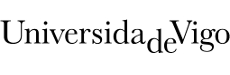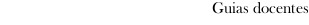Guia docente 2019_20Escuela de Ingeniería Industrial
 Degree in Industrial Technologies EngineeringSubjectsElasticity and additional topics in mechanics of materialsContents
 Topic Sub-topic Fundamentals of elasticity Introduction to the theory of elasticity Stress analysis of elastic solids Strain Stress-strain relationships Two-dimensional elasticity Criteria of failure Saint-Venants failure criterion Trescas failure criterion Von-Mises failure criterion Safety coefficient Bending Non uniform bending: Shear stresses. Zhuravski expression Principal stresses. Stress trajectories Bending and axial load: Normal stresses. Neutral axis Eccentric axial loads Kern of the cross-section Beams of different materials Bending. Statically indeterminate beams General method Settlements in fixed supports Continuous beams Simplifications in symmetric and antisymmetric beams Torsion Definition Coulombs fundamental theory Static torque diagrams Stress and angle of twist Statically indeterminate problems Combined loads Definition Bending and torsion loaded circular shafts Shear center Stress and strain calculation in plane-spatial structures Strain energy and energy methods Strain energy: Axial load/shearing loads/bending/torsion/general expression. Clapeyron's theorem Indirect and direct work MaxwellBetti Reciprocal Theorem. Applications. Castiglianos theorem. Mohr's integrals. Applications. Principle of virtual works. Trusses Definition and general comments Degree of indeterminacy Analytical method of force calculation Pinned joint displacement determination External indeterminacy and internal indeterminacy Structures with rigid joint connections Definition Joint stiffness factor and distribution factor Degree of indeterminacy. Analysis by the stiffness method. Moving loads Influence lines. Definition and general properties.
 Universidade de Vigo            | Rectorado | Campus Universitario | C.P. 36.310 Vigo (Pontevedra) | España | Tlf: +34 986 812 000